|

# 一家七口,蜗居上海60平毛坯房,不动一面墙,两房变三房,这是我见过最走心的改造

十年前，因为大女儿的先天性疾病，杨春艳和于万里夫妇有了生二胎的资格，原本想着，多个宝宝，孩子们长大了彼此还有个伴。

可没想到，750万分之一的概率被他们碰上了，龙凤四胞胎的降生，令这个原本经济就不宽裕的家庭，更加捉襟见肘。八年前，一家七口人租下了这间位于上海闵行的出租屋，使用面积大概60多平方米，装修得很简陋，和毛坯房几乎没两样。

房子里只有两间卧室，朝南的卧室稍大些，四胞胎挤在并排的两张床上；父母则蜗居在只有8平米的朝北次卧；而放了寒暑假回上海的姐姐，通常只能睡在客厅。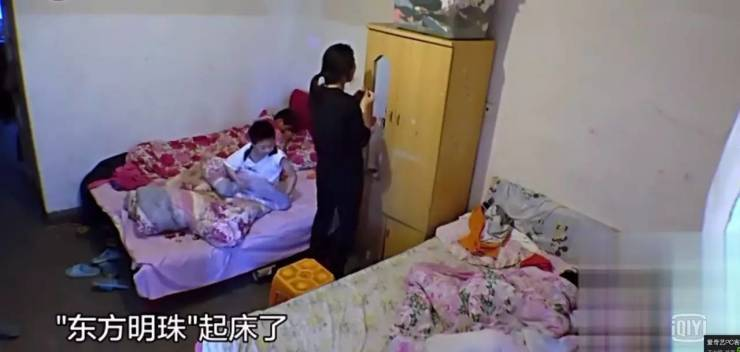十岁的孩子，正是活泼好动的年纪，更别提一家里还有4个！这个要看书，那个要听音乐，还有的要踢足球，玩具、书本全都混着放，谁也找不着谁的。

最痛苦的就是，家里只有一个卫生间……上厕所还得排队……

下面这张漫画，很形象地表现了他们的生活难点

▼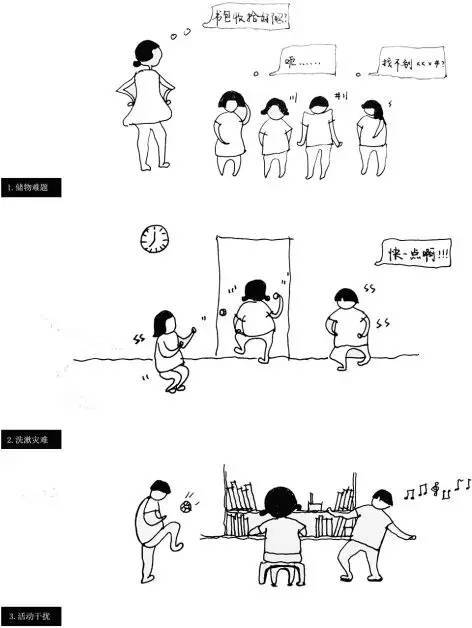前不久，这一家子上了东方卫视的《生活改造家》栏目，上海青年建筑师刘津瑞及其团队，完美地改造了这60㎡空间，一家七口从此有了吃饭、学习、玩乐的空间。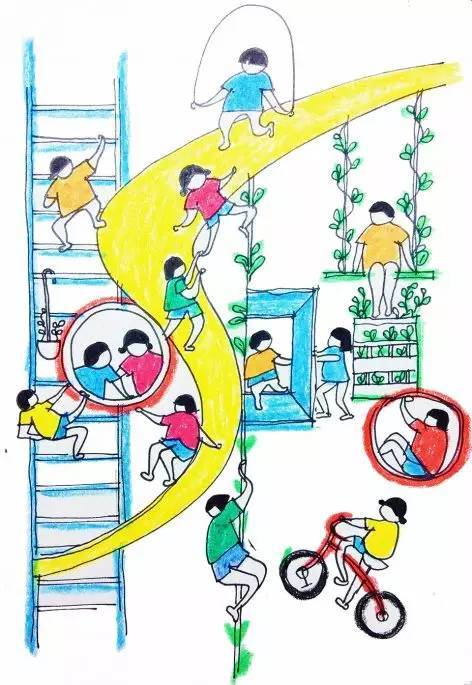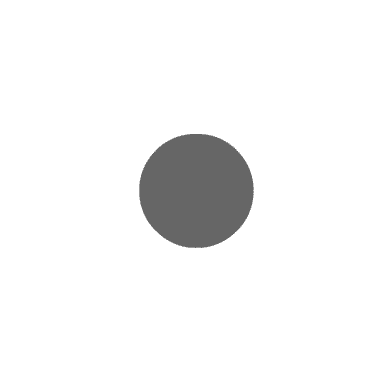先来看下改造效果

▼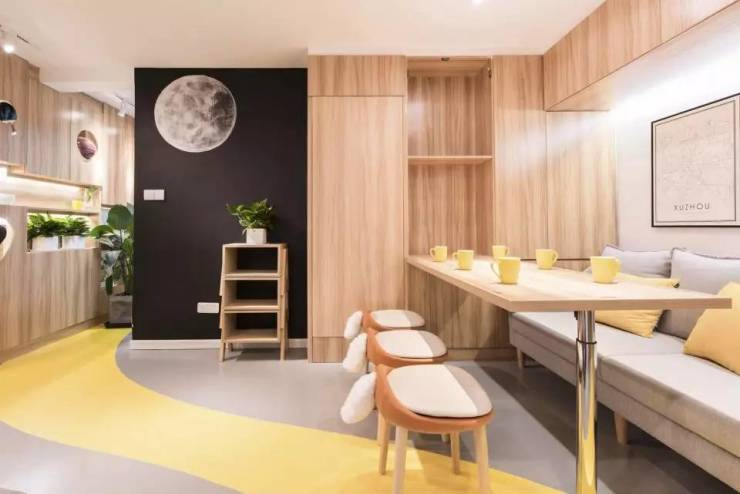房东不准改变房间格局，因此改造并不着重一面墙、一张床、一排柜子的位置，而是力求消解空间的分割，追求流动性和视觉的通透感。

一条好似黄浦江的空气跑道串联起相对孤立的空间。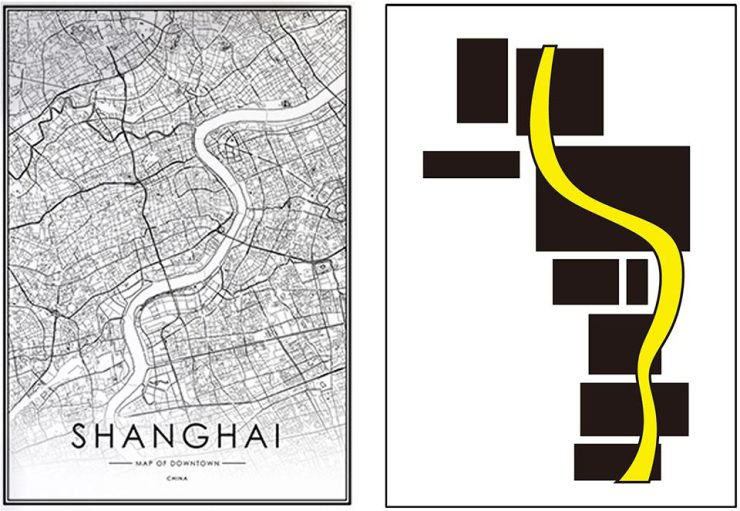空间有限，会将不常用的功能进行压缩、折叠、甚至隐藏。

改造后的七口之家将睡觉、吃饭、储藏等不常用的功能空间进行压缩，而将承载着家庭大部分活动空间的公共空间打通、放大。

尤其表现在厕所和卫生间功能更加精细化，面积精打细算。原先的两件卧室最大化地利用了垂直空间，使得父母、男孩、女孩们都有了自己的独立空间。玄关处树洞状的壁龛可以放下第二天出门的衣物，也可以作为临时的换鞋凳。

卡通图案的高度正好契合儿童的视线高度，希望孩子们都能独立完成出门前的一切准备工作。壁龛正对着的是厨房，壁龛后就是一家人共同进餐的餐桌。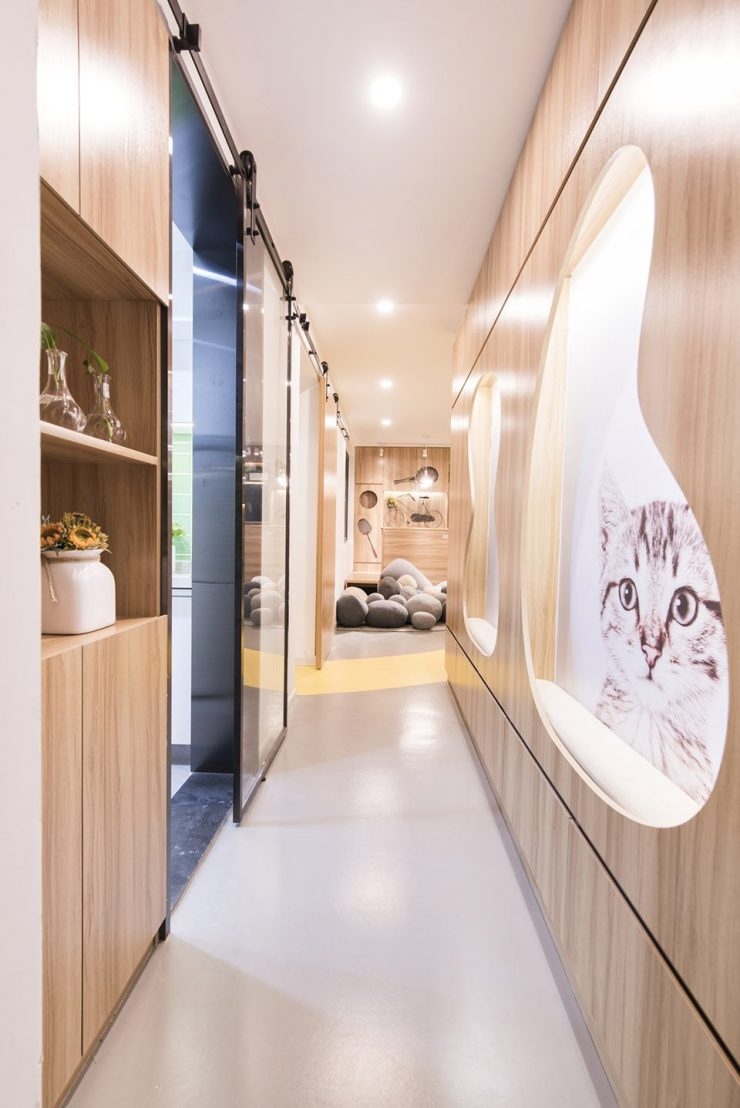厨房被设计成“亲子厨房”，提供了小型设施，诸如，转角小切板、小水槽、小台面等等，这样孩子们都能参与进来。

每天晚上可以和爸爸妈妈一起准备晚餐，到了周末，和妈妈一起烘焙蛋糕甜点……厨房里也存放着最有爱的亲子时光。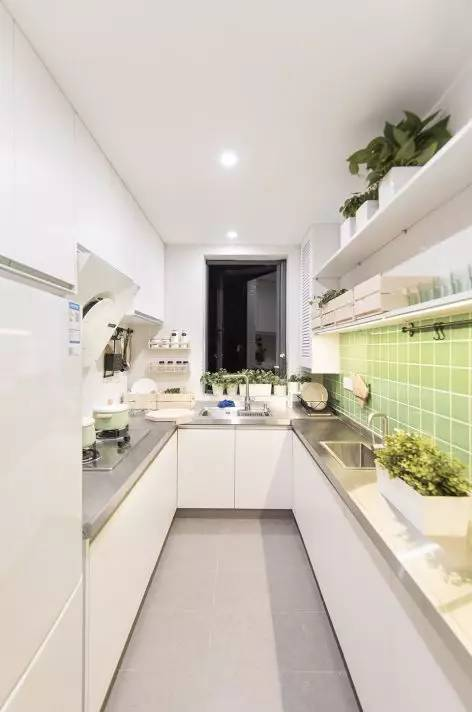按照规划设计，壁龛后是供一家人活动玩乐的公共空间，但当折叠式餐桌放下后，客厅沙发变成了餐椅。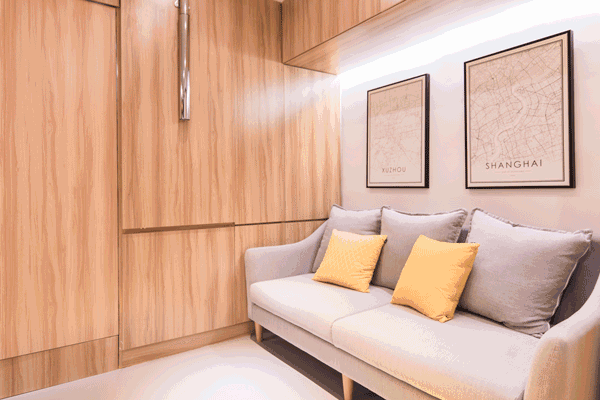长条餐桌收取方便，平日里也足够一家人一同进食。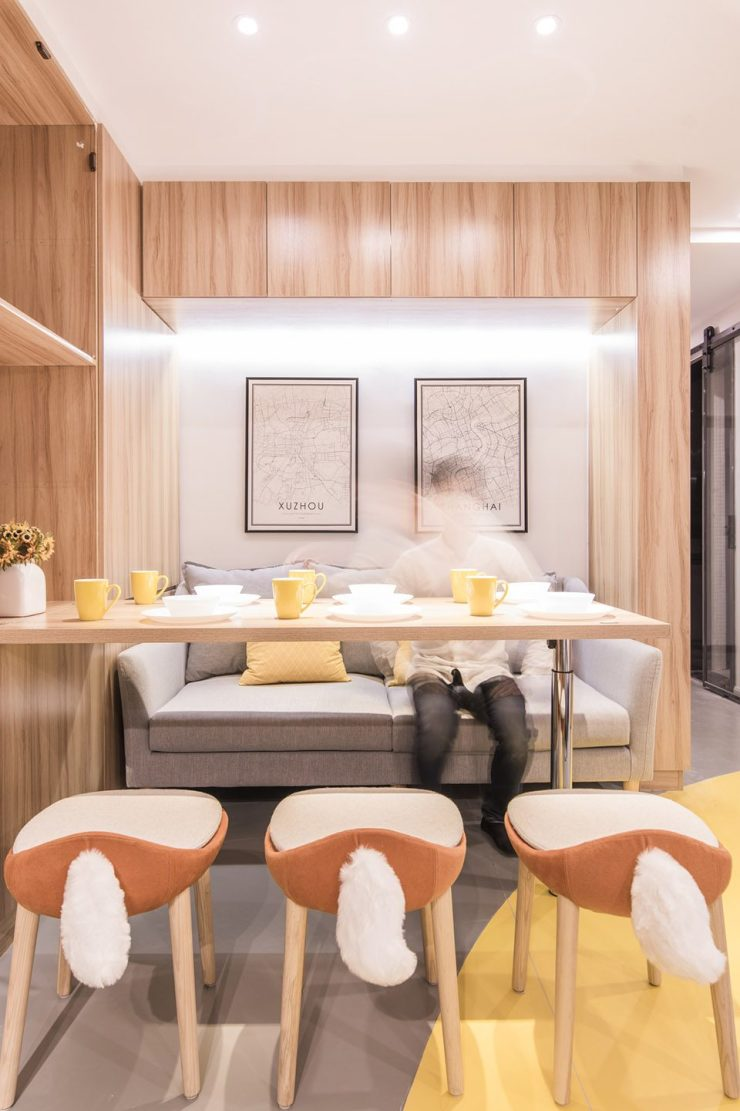▲毛茸茸的木凳尾巴，看着就好想抓住不放！

考虑到4个孩子吵闹起来的爆发力，客厅地面采用的是PVC+消音地毯+鹅卵石抱枕，将对楼下的干扰降到最小。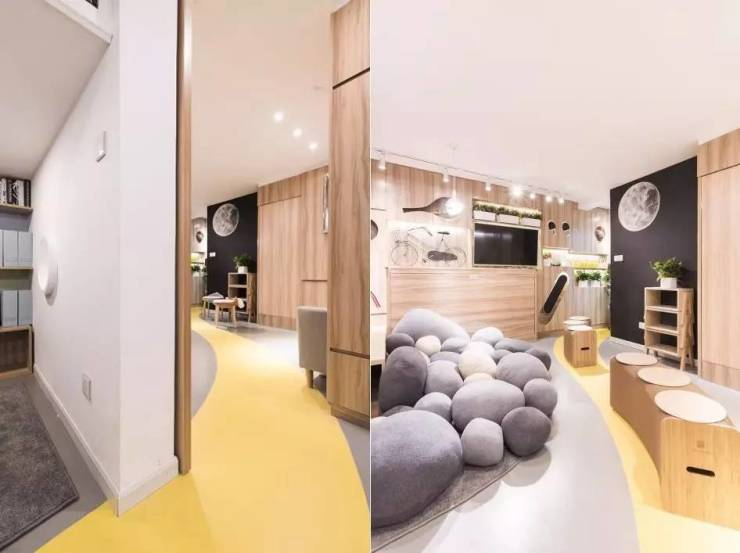犹如黄浦江的黄色跑道从男孩卧室出发，途经客厅，穿过窄过道，连通起各个空间。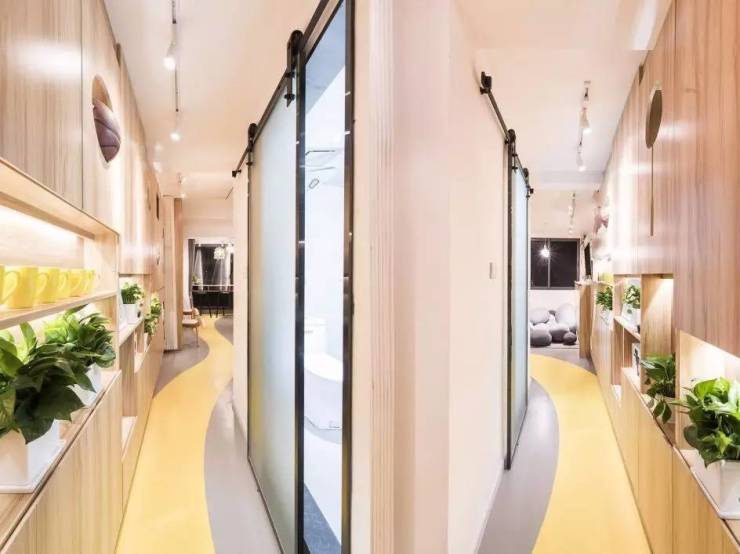在从客厅延伸到主卧的窄柜上，有乒乓球拍、滑板、时钟、相框，有趣而完备的收纳设施，让孩子们从小养成东西用完要放归原位的好习惯。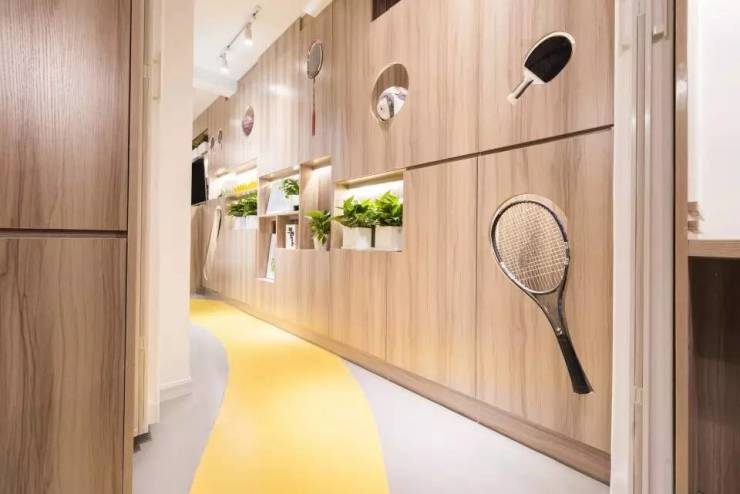卫生间旁装了很时兴的黑板墙，留给孩子们自由想象的空间。再来看看一家七口人各自的生活空间，首先是男孩房。

你可以看到，8㎡的空间被上下分区，睡觉、学习、活动都有了独立的区域。活动空间中的环形座椅，既分割了空间，又是不阻挡视线的公共区域。书架后的山峦图案意喻“书山有路勤为径”，墙面的圆形开洞则有“举头望明月”的意境。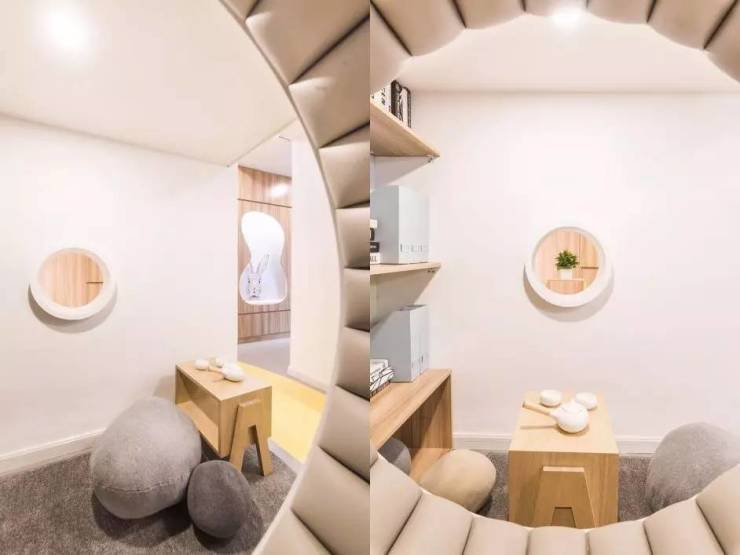接下来是父母的卧室，设计师划分了原先的南向主卧，用移动立柜和折垫床划出父母的睡觉区域。

白天并不占用空间，而到了晚上家庭成员都有了独立房间。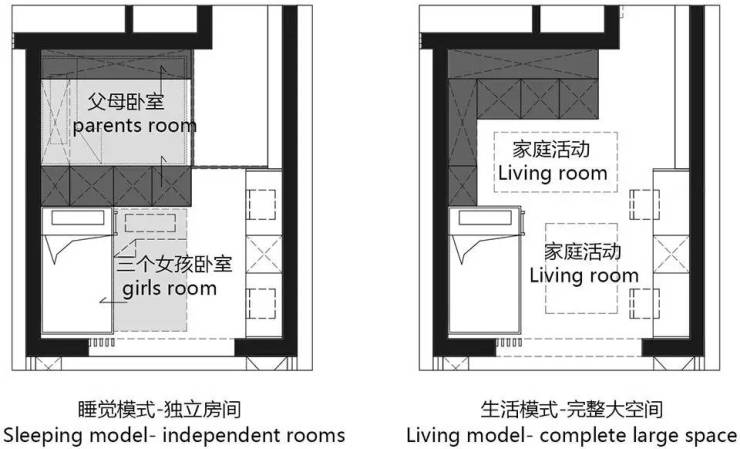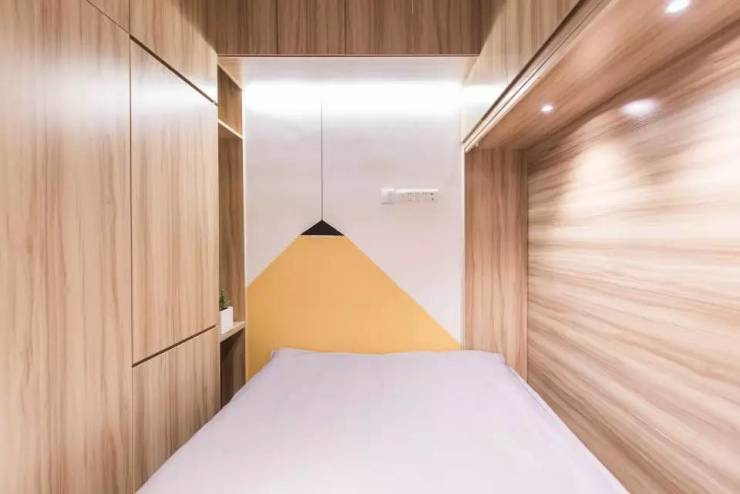而且使用PVC拉帘也能保证两个独立房间的隐私。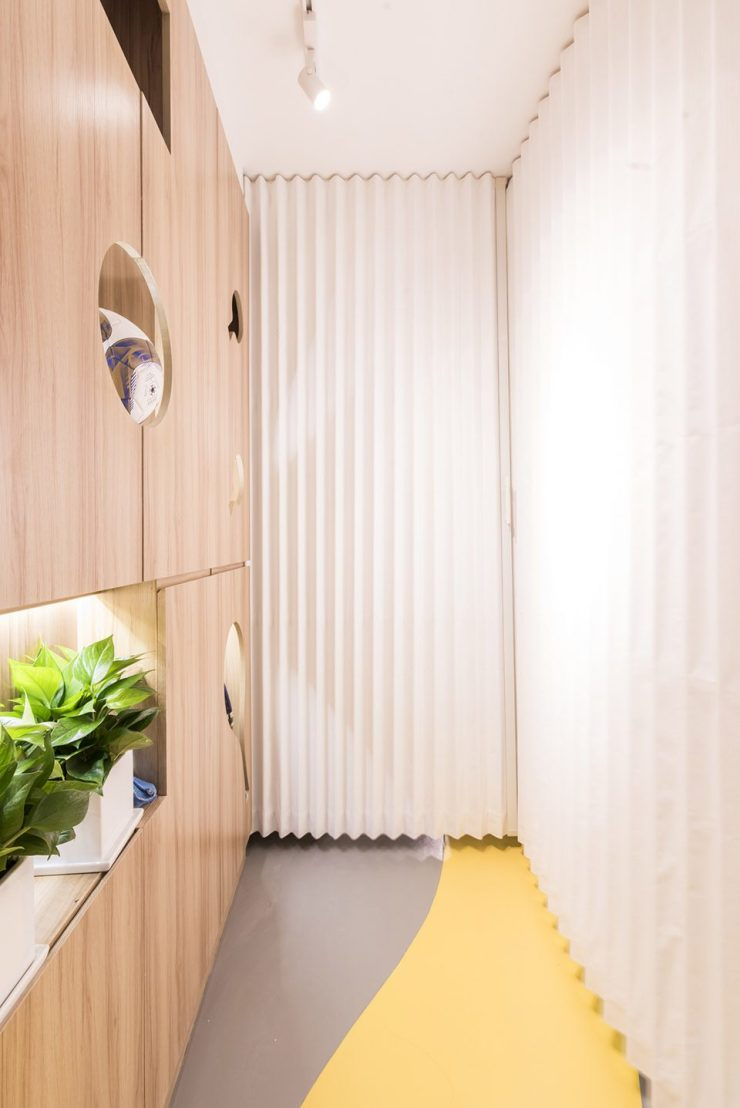考虑到寒暑假，姐姐会回家住，因此留给女孩房的空间最大。

一侧是高低床，一侧是两台书桌，两个小女孩都有了独立的床铺和学习空间，白天将可以动的立柜挪开，活动空间也变大了。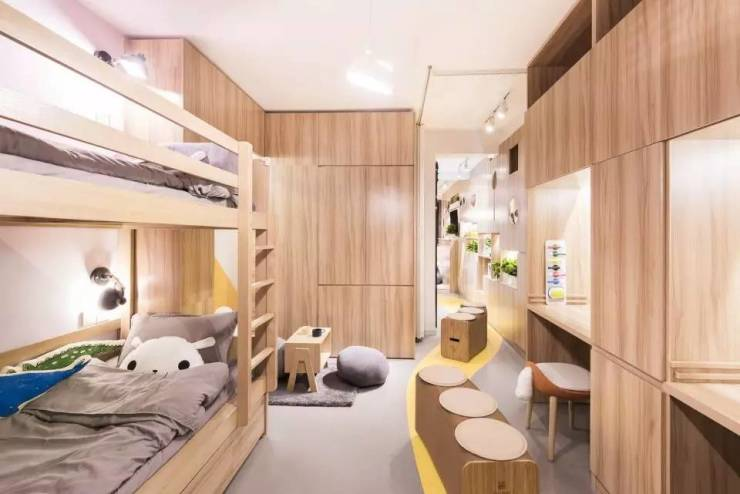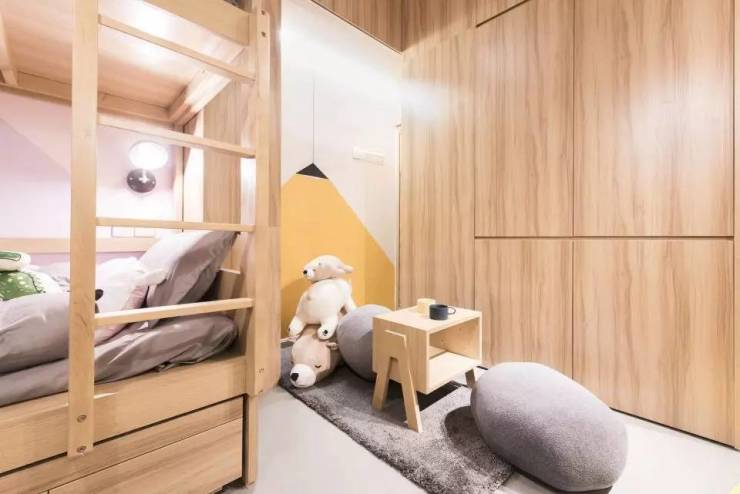设计师在高低床下预留出了第三张床，这样姐姐回上海时，也不用一个人孤零零地窝在客厅了。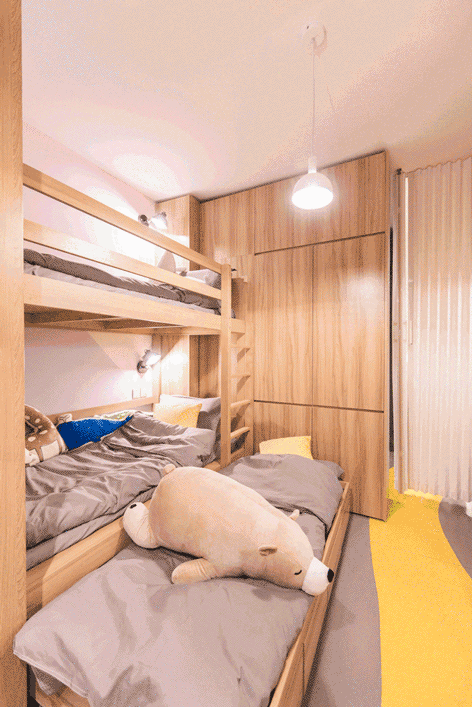走出女孩房就是阳台，阳台上设有条桌和花架，立体植物园里种着小番茄、辣椒、草莓、薄荷、冰草、芹菜等植物。

周末时一群孩子围着条桌研究怎么种植物，从小培养他们热爱自然的心思，颇有几分“童孙未解供耕织，也傍桑阴学种瓜”之感。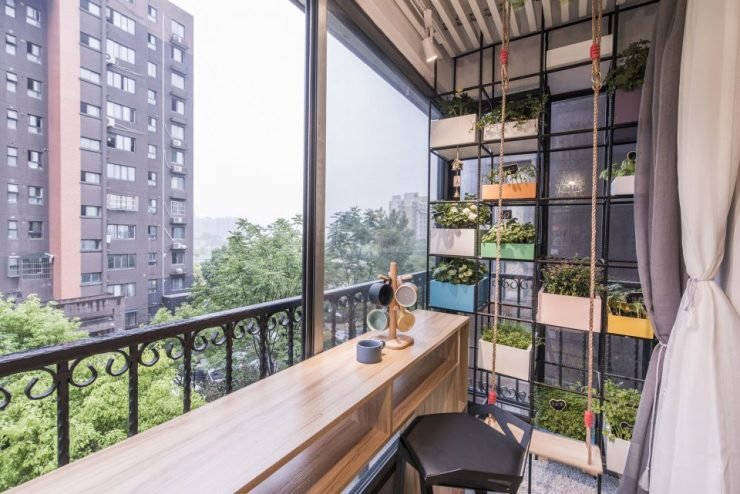能照料好这么多种小作物，想必妈妈也是位兰心蕙质的女子~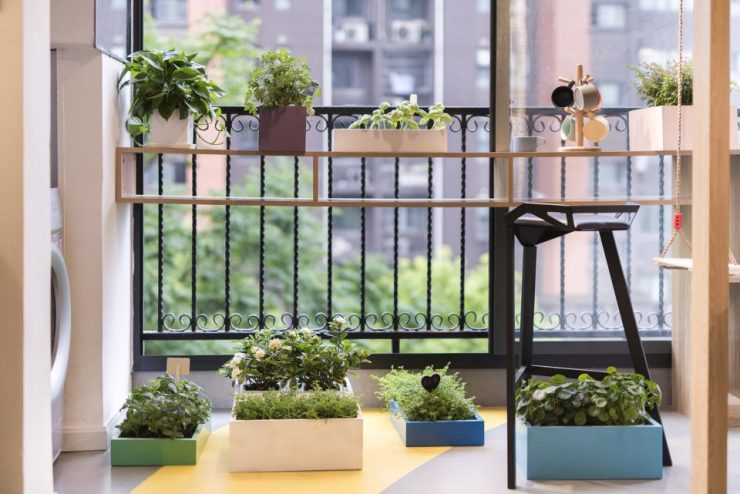之前讲的很多案例中，60多平的屋子通常标配就是两三人而已。

一家七口，一个人也就8.5㎡的空间，说实在的，并不宽敞。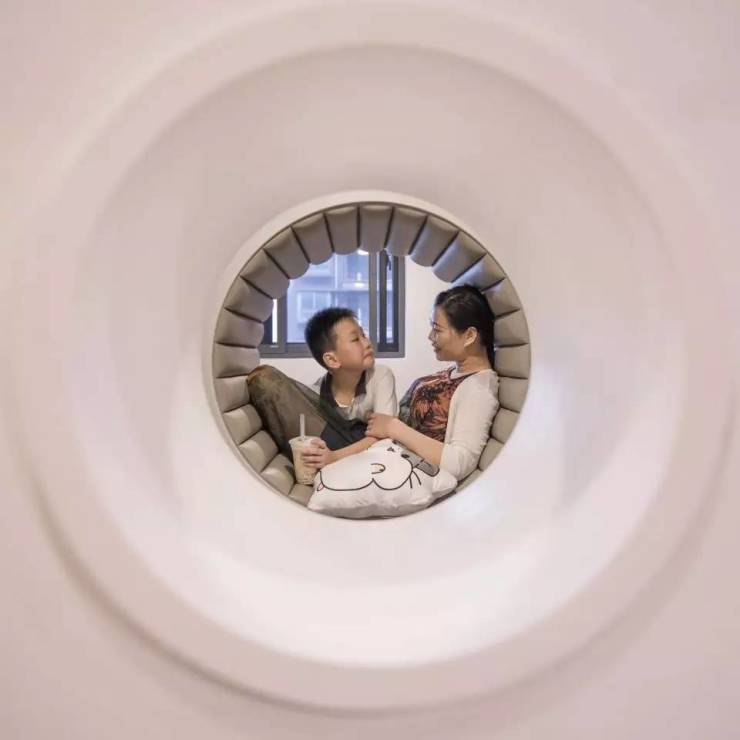但是家庭在意的并不只有这些：一个温柔有趣的空间，能带给孩子更多探索的可能；一个快乐温馨的家庭环境，能让孩子身心更健康的成长。

也许，这才是设计师在改造七口之家时最大的期许！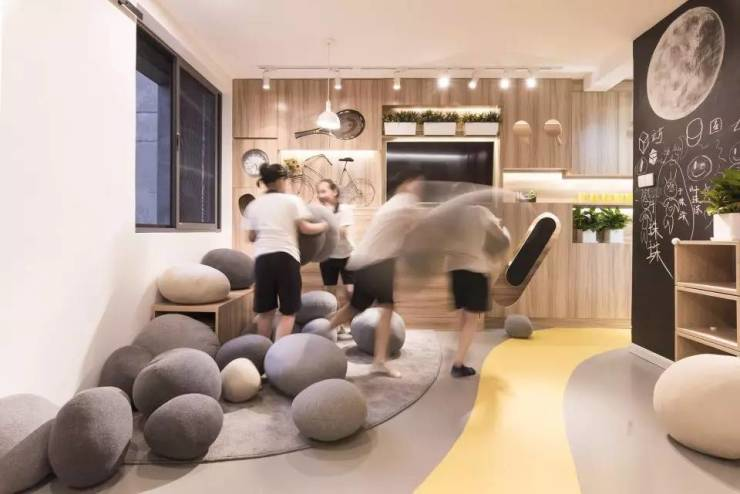`声明：本文由入驻焦点开放平台的作者撰写，除焦点官方账号外，观点仅代表作者本人，不代表焦点立场错误信息举报电话： 400-099-0099，邮箱：jubao@vip.sohu.com，或点此进行意见反馈，或点此进行举报投诉。`A B C D E F G H J K L M N P Q R S T W X Y Z
A - B - C - D - E
• A
• 鞍山
• 安庆
• 安阳
• 安顺
• 安康
• 澳门
• B
• 北京
• 保定
• 包头
• 巴彦淖尔
• 本溪
• 蚌埠
• 亳州
• 滨州
• 北海
• 百色
• 巴中
• 毕节
• 保山
• 宝鸡
• 白银
• 巴州
• C
• 承德
• 沧州
• 长治
• 赤峰
• 朝阳
• 长春
• 常州
• 滁州
• 池州
• 长沙
• 常德
• 郴州
• 潮州
• 崇左
• 重庆
• 成都
• 楚雄
• 昌都
• 慈溪
• 常熟
• D
• 大同
• 大连
• 丹东
• 大庆
• 东营
• 德州
• 东莞
• 德阳
• 达州
• 大理
• 德宏
• 定西
• 儋州
• 东平
• E
• 鄂尔多斯
• 鄂州
• 恩施
F - G - H - I - J
• F
• 抚顺
• 阜新
• 阜阳
• 福州
• 抚州
• 佛山
• 防城港
• G
• 赣州
• 广州
• 桂林
• 贵港
• 广元
• 广安
• 贵阳
• 固原
• H
• 邯郸
• 衡水
• 呼和浩特
• 呼伦贝尔
• 葫芦岛
• 哈尔滨
• 黑河
• 淮安
• 杭州
• 湖州
• 合肥
• 淮南
• 淮北
• 黄山
• 菏泽
• 鹤壁
• 黄石
• 黄冈
• 衡阳
• 怀化
• 惠州
• 河源
• 贺州
• 河池
• 海口
• 红河
• 汉中
• 海东
• I
• J
• 晋中
• 锦州
• 吉林
• 鸡西
• 佳木斯
• 嘉兴
• 金华
• 景德镇
• 九江
• 吉安
• 济南
• 济宁
• 焦作
• 荆门
• 荆州
• 江门
• 揭阳
• 金昌
• 酒泉
• 嘉峪关
K - L - M - N - P
• K
• 开封
• 昆明
• 昆山
• L
• 廊坊
• 临汾
• 辽阳
• 连云港
• 丽水
• 六安
• 龙岩
• 莱芜
• 临沂
• 聊城
• 洛阳
• 漯河
• 娄底
• 柳州
• 来宾
• 泸州
• 乐山
• 六盘水
• 丽江
• 临沧
• 拉萨
• 林芝
• 兰州
• 陇南
• M
• 牡丹江
• 马鞍山
• 茂名
• 梅州
• 绵阳
• 眉山
• N
• 南京
• 南通
• 宁波
• 南平
• 宁德
• 南昌
• 南阳
• 南宁
• 内江
• 南充
• P
• 盘锦
• 莆田
• 平顶山
• 濮阳
• 攀枝花
• 普洱
• 平凉
Q - R - S - T - W
• Q
• 秦皇岛
• 齐齐哈尔
• 衢州
• 泉州
• 青岛
• 清远
• 钦州
• 黔南
• 曲靖
• 庆阳
• R
• 日照
• 日喀则
• S
• 石家庄
• 沈阳
• 双鸭山
• 绥化
• 上海
• 苏州
• 宿迁
• 绍兴
• 宿州
• 三明
• 上饶
• 三门峡
• 商丘
• 十堰
• 随州
• 邵阳
• 韶关
• 深圳
• 汕头
• 汕尾
• 三亚
• 三沙
• 遂宁
• 山南
• 商洛
• 石嘴山
• T
• 天津
• 唐山
• 太原
• 通辽
• 铁岭
• 泰州
• 台州
• 铜陵
• 泰安
• 铜仁
• 铜川
• 天水
• 天门
• W
• 乌海
• 乌兰察布
• 无锡
• 温州
• 芜湖
• 潍坊
• 威海
• 武汉
• 梧州
• 渭南
• 武威
• 吴忠
• 乌鲁木齐
X - Y - Z
• X
• 邢台
• 徐州
• 宣城
• 厦门
• 新乡
• 许昌
• 信阳
• 襄阳
• 孝感
• 咸宁
• 湘潭
• 湘西
• 西双版纳
• 西安
• 咸阳
• 西宁
• 仙桃
• 西昌
• Y
• 运城
• 营口
• 盐城
• 扬州
• 鹰潭
• 宜春
• 烟台
• 宜昌
• 岳阳
• 益阳
• 永州
• 阳江
• 云浮
• 玉林
• 宜宾
• 雅安
• 玉溪
• 延安
• 榆林
• 银川
• Z
• 张家口
• 镇江
• 舟山
• 漳州
• 淄博
• 枣庄
• 郑州
• 周口
• 驻马店
• 株洲
• 张家界
• 珠海
• 湛江
• 肇庆
• 中山
• 自贡
• 资阳
• 遵义
• 昭通
• 张掖
• 中卫

1室1厅1厨1卫1阳台

1
2
3
4
5

0
1
2

1

1

0
1
2
3报名成功，资料已提交审核A B C D E F G H J K L M N P Q R S T W X Y Z
A - B - C - D - E
• A
• 鞍山
• 安庆
• 安阳
• 安顺
• 安康
• 澳门
• B
• 北京
• 保定
• 包头
• 巴彦淖尔
• 本溪
• 蚌埠
• 亳州
• 滨州
• 北海
• 百色
• 巴中
• 毕节
• 保山
• 宝鸡
• 白银
• 巴州
• C
• 承德
• 沧州
• 长治
• 赤峰
• 朝阳
• 长春
• 常州
• 滁州
• 池州
• 长沙
• 常德
• 郴州
• 潮州
• 崇左
• 重庆
• 成都
• 楚雄
• 昌都
• 慈溪
• 常熟
• D
• 大同
• 大连
• 丹东
• 大庆
• 东营
• 德州
• 东莞
• 德阳
• 达州
• 大理
• 德宏
• 定西
• 儋州
• 东平
• E
• 鄂尔多斯
• 鄂州
• 恩施
F - G - H - I - J
• F
• 抚顺
• 阜新
• 阜阳
• 福州
• 抚州
• 佛山
• 防城港
• G
• 赣州
• 广州
• 桂林
• 贵港
• 广元
• 广安
• 贵阳
• 固原
• H
• 邯郸
• 衡水
• 呼和浩特
• 呼伦贝尔
• 葫芦岛
• 哈尔滨
• 黑河
• 淮安
• 杭州
• 湖州
• 合肥
• 淮南
• 淮北
• 黄山
• 菏泽
• 鹤壁
• 黄石
• 黄冈
• 衡阳
• 怀化
• 惠州
• 河源
• 贺州
• 河池
• 海口
• 红河
• 汉中
• 海东
• I
• J
• 晋中
• 锦州
• 吉林
• 鸡西
• 佳木斯
• 嘉兴
• 金华
• 景德镇
• 九江
• 吉安
• 济南
• 济宁
• 焦作
• 荆门
• 荆州
• 江门
• 揭阳
• 金昌
• 酒泉
• 嘉峪关
K - L - M - N - P
• K
• 开封
• 昆明
• 昆山
• L
• 廊坊
• 临汾
• 辽阳
• 连云港
• 丽水
• 六安
• 龙岩
• 莱芜
• 临沂
• 聊城
• 洛阳
• 漯河
• 娄底
• 柳州
• 来宾
• 泸州
• 乐山
• 六盘水
• 丽江
• 临沧
• 拉萨
• 林芝
• 兰州
• 陇南
• M
• 牡丹江
• 马鞍山
• 茂名
• 梅州
• 绵阳
• 眉山
• N
• 南京
• 南通
• 宁波
• 南平
• 宁德
• 南昌
• 南阳
• 南宁
• 内江
• 南充
• P
• 盘锦
• 莆田
• 平顶山
• 濮阳
• 攀枝花
• 普洱
• 平凉
Q - R - S - T - W
• Q
• 秦皇岛
• 齐齐哈尔
• 衢州
• 泉州
• 青岛
• 清远
• 钦州
• 黔南
• 曲靖
• 庆阳
• R
• 日照
• 日喀则
• S
• 石家庄
• 沈阳
• 双鸭山
• 绥化
• 上海
• 苏州
• 宿迁
• 绍兴
• 宿州
• 三明
• 上饶
• 三门峡
• 商丘
• 十堰
• 随州
• 邵阳
• 韶关
• 深圳
• 汕头
• 汕尾
• 三亚
• 三沙
• 遂宁
• 山南
• 商洛
• 石嘴山
• T
• 天津
• 唐山
• 太原
• 通辽
• 铁岭
• 泰州
• 台州
• 铜陵
• 泰安
• 铜仁
• 铜川
• 天水
• 天门
• W
• 乌海
• 乌兰察布
• 无锡
• 温州
• 芜湖
• 潍坊
• 威海
• 武汉
• 梧州
• 渭南
• 武威
• 吴忠
• 乌鲁木齐
X - Y - Z
• X
• 邢台
• 徐州
• 宣城
• 厦门
• 新乡
• 许昌
• 信阳
• 襄阳
• 孝感
• 咸宁
• 湘潭
• 湘西
• 西双版纳
• 西安
• 咸阳
• 西宁
• 仙桃
• 西昌
• Y
• 运城
• 营口
• 盐城
• 扬州
• 鹰潭
• 宜春
• 烟台
• 宜昌
• 岳阳
• 益阳
• 永州
• 阳江
• 云浮
• 玉林
• 宜宾
• 雅安
• 玉溪
• 延安
• 榆林
• 银川
• Z
• 张家口
• 镇江
• 舟山
• 漳州
• 淄博
• 枣庄
• 郑州
• 周口
• 驻马店
• 株洲
• 张家界
• 珠海
• 湛江
• 肇庆
• 中山
• 自贡
• 资阳
• 遵义
• 昭通
• 张掖
• 中卫• 手机• 分享
• 设计
免费设计
• 计算器
装修计算器
• 入驻
合作入驻
• 联系
联系我们
• 置顶
返回顶部